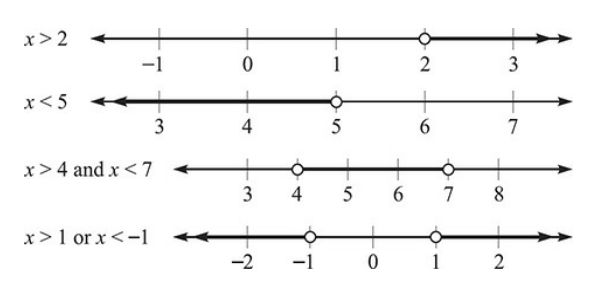# Coordinate Geometry Quiz: Could You Pass?

15 Questions | Total Attempts: 1429SettingsCould you pass this quiz on coordinate geometry? Coordinate geometry describes the connection between geometry and algebra using graphs that are comprised of curves and lines. It gives you the perspective of geometric principles in algebra which allows you to solve problems effectively. Coordinate geometry is a formidable mathematical technique that utilizes algebra for answering geometric problems. This quiz will help you understand coordinate geometry and let you solve some problems to demonstrate your skills. Good luck with the quiz.

• 1.
The points (–4,–8) lies in:
• A.

• B.

• C.

• D.

• 2.
The point (0, –5) lies:
• A.

On the x-axis

• B.

On the y-axis

• C.

• D.

None of the above

• 3.
Ordinate of all the points in the x-axis is:
• A.

0

• B.

1

• C.

–1

• D.

Any natural number

• 4.
If the x-coordinate of a point is zero, then this point lies in the:
• A.

• B.

• C.

On x-axis

• D.

On y-axis

• 5.
If the perpendicular distance of a point P from the x-axis is 7 units and the foot of the perpendicular lies on the negative direction of x-axis, then the point P has:
• A.

Y-coordinate = 7 or –7 only

• B.

Y-coordinate = 7 only

• C.

Y-coordinate = –7 only

• D.

X-coordinate = –7

• 6.
On plotting P (–3, 8), Q (7, –5), R (–3, –8) and T (–7, 9) are plotted on the graph paper, then point(s) in the third quadrant are:
• A.

P and T

• B.

Q and R

• C.

Only R

• D.

P and R

• 7.
If the coordinates of the two points are P (–7, 5) and Q (–6, 9), then (abscissa of P) – (abscissa of Q) is
• A.

–3

• B.

1

• C.

–2

• D.

–1

• 8.
Abscissa of a point is positive in:
• A.

• B.

• C.

• D.

• 9.
The point whose ordinate is 8 and lies on y-axis:
• A.

(0, 8)

• B.

(8, 0)

• C.

(5, 8)

• D.

(8, 5)

• 10.
The coordinates of any point on the y-axis are of the form (0, k), where |k| is the distance of the point from the:
• A.

Y-axis

• B.

X-axis

• C.

(0, 1)

• D.

(1, 0)

• 11.
The mirror of a point (3, 4) on y-axis is:
• A.

(3, 4)

• B.

(–3, 4)

• C.

(3, –4)

• D.

(–3, –4)

• 12.
The distance of the points (5, 0) and (–3, 0) along y-axis is:
• A.

–3

• B.

5

• C.

0

• D.

2

• 13.
The perpendicular distance of a point P (5, 8) from the y-axis is:
• A.

5

• B.

8

• C.

3

• D.

13

• 14.
A point (x + 2, x + 4) lies in the first quadrant, the mirror image for which for x-axis is (5, –7). What is the value of x?
• A.

(–5, –7)

• B.

(–5, 7)

• C.

(5, –7)

• D.

(5, 7)

Related TopicsBack to top